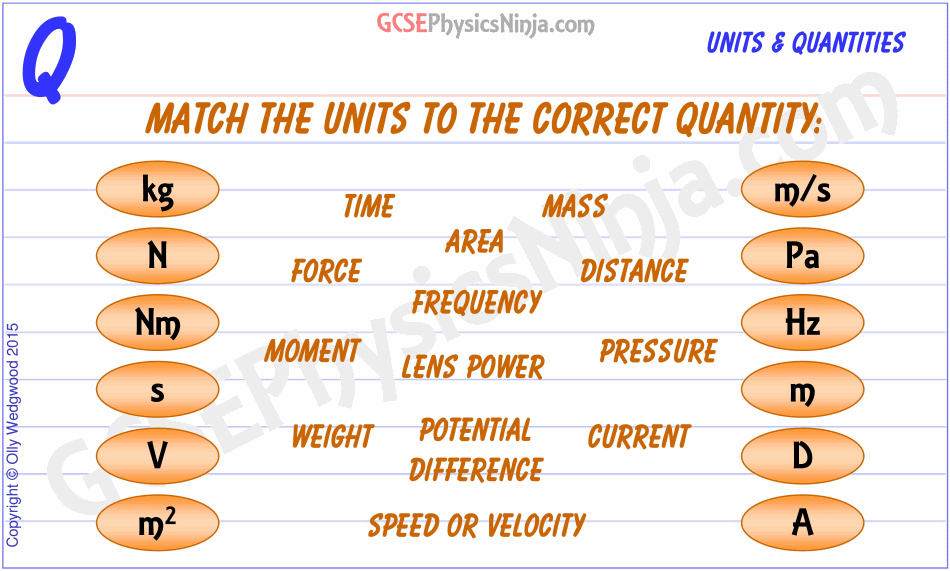# 1. Units and quantities-

``` ```

## The Units of Physics

The oil that keeps the calculation engines of physics running smoothly is the system of units used in the measurement of different quantities. Most countries have adopted the S.I. Unit system (The International System of Units) and this is made up of several 'base units' which represent quanties that we can measure, and other combinations of those base units. It's important that we're able to connect the symbols of these units with the quantities that they represent.

First of all, let's identify the four base units that we're going to look at in this flashcard and then we'll take a look at other derived units.

### The Base Units (there are a few more, but these are the main ones you need to know for GCSE)

• 'Kg' is the unit symbol for kilogram, which is the quantity called 'mass'. Note that 'kilo' is a prefix meaning 'a thousand'.
• 'm' is the unit symbol for metres, which is the quanity called length, distance or displacement.
• 'A' is the symbol for Ampere (or amp for short). This unit represents 'current' which is the flow of charge in an electrical circuit.
• 's' is the unit symbol for seconds and represents the quantity 'time'.

Several derived units are made up of the above base units - these are explained in the video below.

GCSE Physics keywords: unit, quantity, symbols, kilogram, metre, second, amp

Course overview